# Coherent analytic sheaf

(diff) ← Older revision | Latest revision (diff) | Newer revision → (diff)
A coherent sheaf ofmodules on an analytic space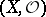. A spaceis said to be coherent ifis a coherent sheaf of rings. Any analytic space over an algebraically closed field is coherent. The most important examples of a coherent analytic sheaf on such a spaceare a locally free sheaf (that is, an analytic sheaf locally isomorphic to the sheaf) and also the sheaf of ideals of an analytic set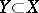, that is, the sheaf of germs of analytic functions equal toon, .
If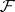is a coherent analytic sheaf on a complex-analytic space, then the space of its sections,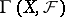, is endowed with a natural topology turning it into a Fréchet space whenis separable. For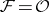, this topology is the same as the topology of uniform convergence of analytic functions on compacta. In this case,becomes a Fréchet sheaf, that is, for arbitrary open setsthe restriction mapping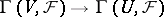is continuous. An analytic homomorphism of coherent sheaves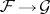induces a continuous linear mapping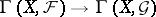. Ifis a coherent analytic sheaf onandis a submodule of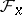,, then the submodule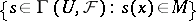is closed infor any neighbourhoodof. The cohomology spaces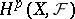also have a natural topology, which is not, in general, separable for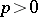(they are quotient spaces of Fréchet spaces) , .
Coherent analytic sheaves were introduced in connection with problems in the theory of analytic functions on domains in(see , ). Later they and their cohomology became a fundamental tool in the global theory of analytic spaces. Criteria for the vanishing of cohomology with values in a coherent analytic sheaf (cf. Kodaira theorem; Ample vector bundle; Stein space) as well as criteria for its finiteness and separability (see Finiteness theorems in the theory of analytic spaces) play an important role in this theory.